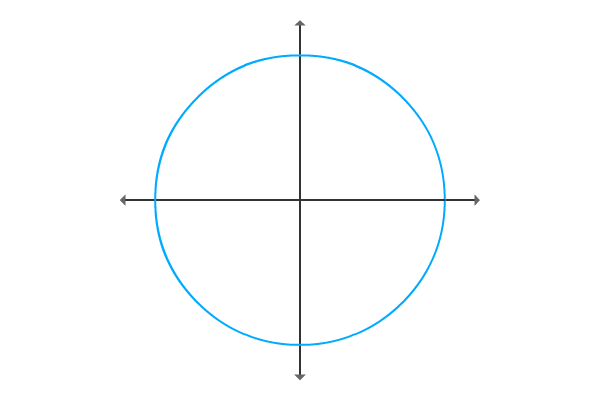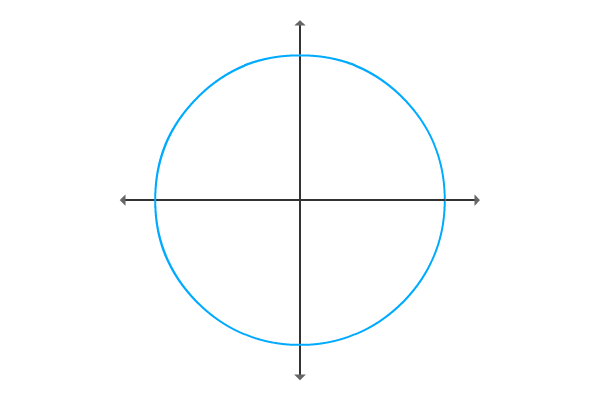# Csc of negative angle

A mathematical relation of Cosecant of negative angle with Cosecant of positive angle is called Cosecant of negative angle identity.

## Formula

$\csc{(-\theta)} \,=\, -\csc{\theta}$

### Proof

The mathematical relation between Cosecant of positive angle and Cosecant of negative angle is derived mathematically in trigonometry by geometrical method.

#### Construction of triangle with positive angle$\Delta POQ$ is a right angled triangle and its construction is done geometrically with a positive angle theta. So, express cosecant of positive angle in terms of ratio of lengths of the corresponding sides.

$\csc{\theta} \,=\, \dfrac{OP}{PQ}$

However, this triangle is construction in first quadrant. Therefore, the lengths of both adjacent and opposite sides are positive and denoted by $x$ and $y$ respectively.

$\implies$ $\csc{\theta} \,=\, \dfrac{\sqrt{x^2+y^2}}{y}$

#### Construction of triangle with negative angleSimilarly, construct same triangle with negative angle but the magnitude of this triangle should be same. Therefore, the angle of $\Delta ROQ$ is negative theta, represented by $–\theta$.

Now, express the trigonometric ratio co-secant in terms of ratio of the lengths of associated sides.

$\csc{(-\theta)} \,=\, \dfrac{OR}{RQ}$

The length of opposite side will be negative because of construction of triangle with negative angle. Geometrically, the length of the opposite side will be $–y$ but the length of adjacent side does not chance.

$\implies$ $\csc{(-\theta)} \,=\, \dfrac{\sqrt{x^2+y^2}}{-y}$

$\implies$ $\csc{(-\theta)} \,=\, -\dfrac{\sqrt{x^2+y^2}}{y}$

#### Comparing sine functions

Now, investigate the relation between cosecant of positive angle and cosecant of negative angle by comparing them.

$\csc{\theta} \,=\, \dfrac{\sqrt{x^2+y^2}}{y}$

$\csc{(-\theta)} \,=\, -\dfrac{\sqrt{x^2+y^2}}{y}$

The comparison of both cosecant functions disclose that cosecant of negative angle equals to negative of cosecant of positive angle.

$\csc{(-\theta)} \,=\, -(\csc{\theta})$

$\,\,\, \therefore \,\,\,\,\,\,$ $\csc{(-\theta)} \,=\, -\csc{\theta}$

This negative identity is called cosecant of negative angle identity and frequently used as a formula in trigonometric mathematics.

Latest Math Topics
Jun 26, 2023
Jun 23, 2023

###### Math Questions

The math problems with solutions to learn how to solve a problem.

Learn solutions

Practice now

###### Math Videos

The math videos tutorials with visual graphics to learn every concept.

Watch now

###### Subscribe us

Get the latest math updates from the Math Doubts by subscribing us.